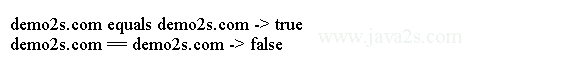# Java Tutorial - Java String

The String class represents character strings. A quoted string constant can be assigned to a String variable.

## Java String Literal

String literals in Java are specified by enclosing a sequence of characters between a pair of double quotes. In Java strings are actually object types.

The following code declares String type variable with Java String literal.

```public class Main{
public static void main(String[] argv){
String str = "this is a test from java2s.com";
System.out.println(str);
}
}
```

The output: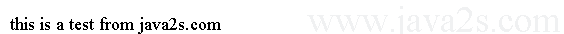## Java String Concatenation

You can use `+` operator to concatenate strings together.

For example, the following fragment concatenates three strings:

```
public class Main {
public static void main(String[] argv) {
String age = "9";
String s = "He is " + age + " years old.";
System.out.println(s);
}
}
```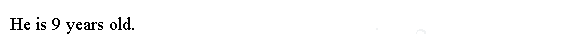## Example

The following code uses string concatenation to create a very long string.

```
public class Main {
public static void main(String args[]) {
//from   www. j a  v  a2 s.c om
String longStr = "A java 2s.      com" +
"B j a v a 2 s . c o m                     " +
"C java                 2s.com" +
"D java2s.com .";

System.out.println(longStr);
}
}
```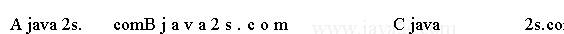## Example 2

You can concatenate strings with other types of data.

```
public class Main {
public static void main(String[] argv) {
int age = 1;
String s = "He is " + age + " years old.";
System.out.println(s);
}
}
```

The output: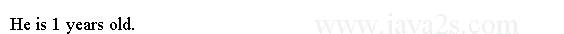## Example 3

Be careful when you mix other types of operations with string concatenation. Consider the following:

```
public class Main {
public static void main(String[] argv) {
String s = "four: " + 2 + 2;
System.out.println(s);
}
}
```

This fragment displays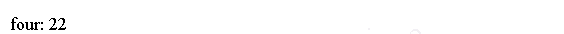rather than the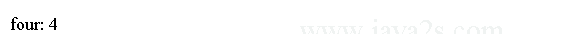To complete the integer addition first, you must use parentheses, like this:

```String s = "four: " + (2 + 2);
```

Now s contains the string "four: 4".

## Java String Escape

The escape sequences are used to enter impossible-to-enter-directly strings.

For example, "`\"`" is for the double-quote character. "`\n`" for the newline string.

For octal notation, use the backslash followed by the three-digit number. For example, "`\141`" is the letter "a".

For hexadecimal, you enter a backslash-u (`\u`), then exactly four hexadecimal digits. For example, "`\u0061`" is the ISO-Latin-1 "`a`" because the top byte is zero. "`\ua432`" is a Japanese Katakana character.

## Escape List

The following table summarizes the Java String escape sequence.

Escape SequenceDescription
\dddOctal character (ddd)
\'Single quote
\"Double quote
\\Backslash
\rCarriage return
\nNew line
\fForm feed
\tTab
\bBackspace

Examples of string literals with escape are

```"Hello World"
"two\nlines"
"\"This is in quotes\""
```

The following example escapes the new line string and double quotation string.

```public class Main {
public static void main(String[] argv) {
String s = "java2s.com";
System.out.println("s is " + s);
//w w w.j av a 2s .com
s = "two\nlines";
System.out.println("s is " + s);

s = "\"quotes\"";

System.out.println("s is " + s);

}
}
```

The output generated by this program is shown here:## Example 4

Java String literials must be begin and end on the same line. If your string is across several lines, the Java compiler will complain about it.

```
public class Main {
public static void main(String[] argv){
String s = "line 1
line 2
";

}
}
```

If you try to compile this program, the compiler will generate the following error message.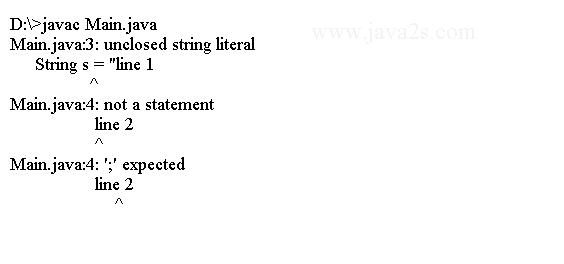## equals() vs ==

`equals( )` method and the `==` operator perform two different operations. `equals( )` method compares the characters inside a String object. The `==` operator compares two object references to see whether they refer to the same instance.

The following program shows the differences:

```
public class Main {
public static void main(String args[]) {
String s1 = "demo2s.com";
String s2 = new String(s1);

System.out.println(s1 + " equals " + s2 + " -> " + s1.equals(s2));
System.out.println(s1 + " == " + s2 + " -> " + (s1 == s2));
}
}
```

Here is the output of the preceding example: